# 17: Is

In this lesson, we give more detail about how Python stores lists. Copying and comparing lists can have unexpected consequences. We will explain how Python works internally, so that you can understand and avoid these mistakes. The general issue is that several different variables can "point to" or "refer to" the same list. Towards the end of the lesson we describe the "`is`" operator which tells whether two variables really point to exactly the same list.

# Example

Let's say we want a code fragment to convert a list of lengths in inches called `oldSize`, to a list of the same lengths in centimeters called `newSize`. One way we might try to do this is to use the line `newSize = oldSize` to make a copy, and then go through and change all the values:

```oldSize = ["letter", 8.5, 11]   # size of paper in inches
newSize = oldSize               # make a copy?
newSize = newSize*2.54    # convert to cm
newSize = newSize*2.54    # convert to cm```
Now let's see if this actually works. If you print `newSize` then it gives `["letter", 21.59, 27.94]` as expected. What is important to notice is that when we print `oldSize`, the values of `oldSize` have changed! We illustrate below:

Example
The second line of output is not what we expect!

We will now give a detailed explanation of exactly what happened, using diagrams. The main problem is that `newSize = oldSize` didn't really copy the whole list: it just copied a reference (arrow) to the same list.

Click on the slide titles to change tabs.

Memory
We'll use a table to represent the variables and values in Python's memory. For example, after running the code

```city = "Moose Factory"
population = 2458
employer = city```
this table (with the black border) shows what Python's memory looks like: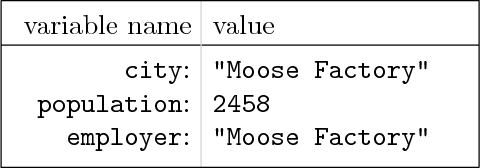The first variable's name is `city` and its value is the string `"Moose Factory"`. The second variable's name is `population` and its value is the integer `2458`. The third variable's name is `employer` and its value is the string `"Moose Factory"`.
Lists in memory
Next, we show what a list looks like in memory. For example, take the code fragment

`myList = ["Moose Factory", 2458]`
This will just create one variable, named `myList`. A list is created, and the value of `myList` is set equal to "point" or "refer" to that list. We represent the list using a box, and the values of the list's entries are shown inside the box next to their corresponding indices. The list is shown in blue.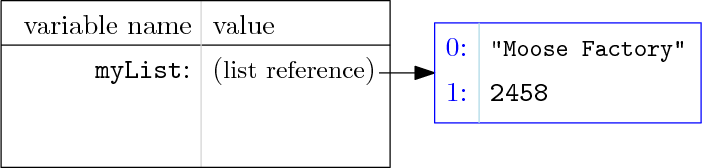The arrow shows that `myList` refers to this new list. The element at index 0 of the list is the string `"Moose Factory"`, and the element at index 1 is the integer `2458`. For example, if you `print(myList)` then Python will output `2458`.
Replacing a list value
Now we add one more line to the previous example, just for illustrative purposes. A baby is born, so we run the code fragment

```myList = ["Moose Factory", 2458]
myList = myList+1```
Python calculates `2458+1` which equals `2459`, and this replaces the value at index 1 of the list. After the update, we have the diagram shown below.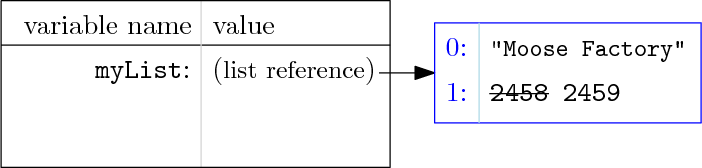(The crossed-out 2458 is shown just to emphasize the change.)
`oldSize` and `newSize`
Now we get back to the main example. The first line was

`oldSize = ["letter", 8.5, 11]`
and so Python's memory looks like the diagram below after this line is executed. We created a list of length 3.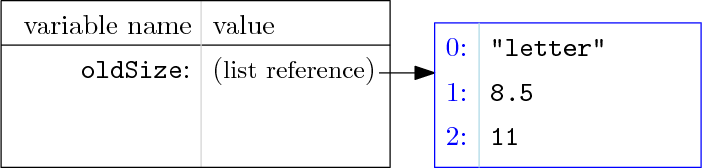Main problem
In our program, the second line is

`newSize = oldSize`
and the most important point in the lesson is here: `=` does not duplicate the list! Instead, it just creates a new reference to the same list. This is illustrated by two different arrows pointing to the exact same box.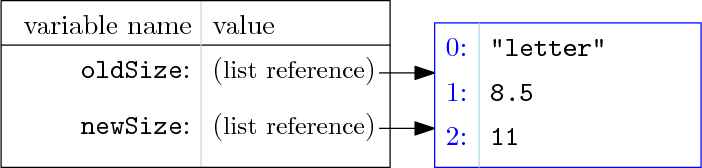As the diagram shows, we have two variables, which both refer to the same list.
Updating
Next, when the program reaches the line

`newSize = newSize*2.54`
Python looks at `newSize`, looks up the value of its index 1 (8.5) and multiplies it by 2.54, and then replaces the value, as shown. However, since `oldSize` referred to the same list, a side effect is that we also affected what `oldSize` refers to!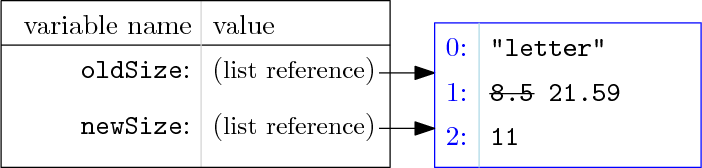(Again 8.5 is shown just to emphasize the change.)
Result
The next line is similar,

`newSize = newSize*2.54`
which affects the other value in the list. After this line executes, Python's memory looks like the diagram shown below.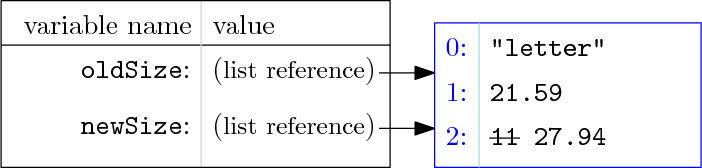Now when we print either `newSize` or `oldSize`, Python outputs `["letter", 21.59, 27.94]`.To just see the pictures without the commentary, run the same code in the visualizer.Actually, every value in Python is an object living in a specific chunk of memory, and every variable is just a reference/pointer/arrow. E.g., in the first slide, memory only really contains one "Moose Factory" with two arrows to it. Because number and string objects are "immutable" we draw them without arrows in these lessons, but behind the scenes, Python treats all data uniformly. Read more here.

# "Deep" Copying a List in Python

In the above example, we use `=` to create a second pointer/reference/arrow to an existing, list, which is usually called a "shallow" copy. Although it is sometimes useful to do this, here it is not what we wanted. Instead, we wanted to create an entirely new copy of that list. How can this be done?

We'll give three solutions. They are all pretty much equivalent, but each one will teach you a new fact about Python, so all three are worth reading.

## Method 1, using `[:]`

Example
Copying with `[:]`

Above, we demonstrate that `newList = oldList[:]` creates a real copy of the old list. Although this syntax looks strange, it is a relative of something we already saw. In the string lesson, we introduced a way of extracting a substring: `string[first:tail]` returns the substring starting with index `first` and ending with index `tail-1`. We mentioned it can also apply to create sublists of lists. In addition,

• if you omit `first`, then it takes the default value of `0`;
• if you omit `tail`, then it takes the default value of `len` (the length of the list/string).

So what really happens is that `oldList[:]` is creating a new sublist, but containing all of the same data as the original list, so it is a new copy.

## Method 2, using `copy.copy()`

There is a module called `copy`, which contains several methods related to copying. The simplest one is `copy.copy(L)`: when you call this on a list `L`, the function returns a real copy of `L`.

Example
Copying with `copy.copy`

The `copy.copy()` function also works for other kinds of objects, but we won't discuss them here.

## Method 3, using `list()`

The last way of making a real copy is using the list() function. Ordinarily, `list()` is used to convert data from other types to the `list` type. (For example `list("hello")` converts the string "hello" to a 5-element list, each element being one character.) But if you try to convert something that is already a list into a list, it just makes a copy.

Example
Copying with `list()`

## Lists as Arguments

Notice that because of the way that lists work, any function which accepts a list as an argument can actually change the contents of the list. (You saw this already in the `replace` exercise.)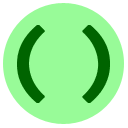What number is output by the following program?

`def func(list):    list = list + list    list = list + listdata = [3, 4]func(data)print(data*data)`
Correct! Inside `func`, we change the element at index 0 to 7, and the element at index 1 to 7+4=11. So we print 7*11.

## Comparing lists using `is`

When are two list variables `L1` and `L2` equal? There are two different ways that we can interpret this question:

• Same Identity: Are `L1` and `L2` pointing/referring to the exact same list object?
• Same Values: Are the contents of list `L1` equal to the contents of list `L2`?

It turns out that in Python, the standard equality operator `==` has the Same Values meaning, as the following example shows.

Example
The meaning of `==`

To test for Same Identity, Python has the `is` operator. We use this operator the same way as `==`: the syntax

`«list1» is «list2»`

returns `True` if the lists refer to the same list, and `False` if they refer to different lists (even if they have the same contents).

Example
The meaning of `is`How many times does `True` show up in the output of this program? (Draw a diagram to help keep track.)

`list1 = [9, 1, 1]list3 = list(list1)list2 = list1list4 = list3[:]list1 = 4list5 = list1print(list1 is list2, list2 is list3, list3 is list4, list4 is list5)print(list1 == list2, list2 == list3, list3 == list4, list4 == list5)`
Correct! The output is
`True False False FalseTrue False True False`You should not use `is` with strings or numbers because == already correctly tests equality, and the behaviour of `is` is hard to predict on strings and numbers.

## Nested lists

We have already given most of the important information, but there is one other common situation worth mentioning. As mentioned in the previous lesson, a nested list is a list within another list, for example

`sample = [365.25, ["first", 5]]`

The outer list, which is what `sample` refers to, has two elements; the element at index 0 is a float and the element at index 1 is the inner list. The inner list is `["first", 5]`. (You can have more levels of nesting too.) Once you start using nested lists, keep in mind:

• Applying the three methods above to `sample` will copy the outer list, but not the inner list. So `copy(sample) is sample`, meaning the copy still has a reference to part of the original list. This probably was not what you wanted. If you want to make a real copy at all levels, use `copy.deepcopy()`.
• Testing nested lists with `==` is pretty intuitive: Python recursively calls `==` on each list element. For example `[[1, 2], 3]==[[1, 2], 3]` is `True`, and `[[1, 2], 3]==[1, 2, 3]` is `False` since the first elements are different (`[1, 2] != 1`).

Example
`deepcopy` and recursive equality

You have now completed this lesson! The following extra material is optional.

## Tuples: `("immutable", "lists")`

We mentioned above that when you call a function on a list, it can alter the list. Sometimes you want to make this impossible! A solution in Python is to create tuples, which are the same as lists except they can never be changed. We say lists are "mutable" and tuples are "immutable". (Strings and numbers are also immutable.) This can be a useful way to prevent any programming errors from altering lists that should stay unchanged. Tuples are almost identical to lists except that they use round parentheses `()` instead of square brackets `[]`. You can convert between tuples and lists using `tuple()` and `list()`.

Example
Tubular tuples

## To `∞` and Beyond: Self-Containment

It is possible to have a list that contains itself! Simply make a list, and then redirect one of its elements to point back to the whole list:

Example
A circular reference

Notice that Python's output engine is smart enough to recognize that the list loops back on itself: it prints "`...`" instead of printing all of `L` again, to avoid an infinite loop.